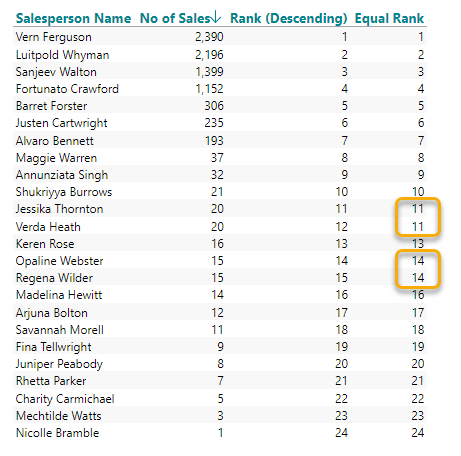# Equal Ranking

The built-in TARGIT calculations 'Rank (Descending)' and 'Rank (Ascending' are able to provide ranking values for dimension members according to a selected measure, e.g., 'No of Sales'. However, the ranking will always be a continuos list of integer numbers - even if two or more dimensions members have identical measure values.

To treat two or more dimension members with identical measure values as having the same rank, you will need to apply a workaround calculation. For this workaround to work, it is required that the crosstab is sorted, descending or ascending, according to the measure value.

In the sample screenshot I have sorted the crosstab (descending) by the 'No of Sales' measure. For reference, I have added the 'Rank (Descending)' calculation from the list of predefined TARGIT calculations. Finally, I have added the 'Equal Rank' calculation as a calculated column:

`if sum(d1, 0, m1) = sum(d1, -1, m1, 0) then sum(c2, -1, m1) else sum(c1, 0, m1)`